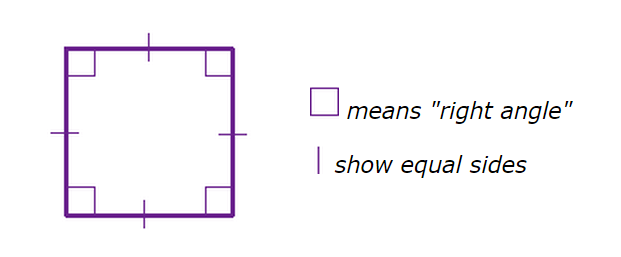Problems
415 - Area of Square

Area of Square

Time Limit: 1 sec

The Problem

A Square is a flat shape with 4 equal sides and every angle is a right angle (90°)Characteristic of Square

1. All sides are equal in length

2. Each internal angle is 90°

3. Opposite sides are parallel

The Area of square is the side length(a) squared.

Area = a2 = a x a

Write a program that read an integer number which is the side length (a) of Square, your task is to find the area of the Square.

The Input

Input file contain a series of line, each line contains only one integer numbers a , which is the side length of square (0<a<=1000). Input is terminated by EOF.

The Output

For each line of input, output will show the one integer number which is the area of the square in a separate line.

Sample Input

10

20

5

Sample Output

100

400

25

Problem Setter: Shahin ShamS